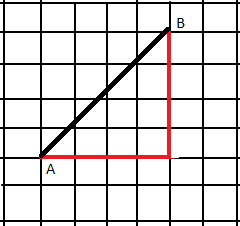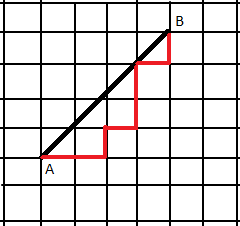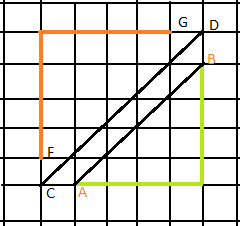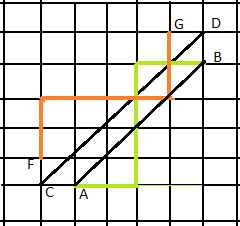# Dyck Words of given length

Given an integer n, the task is to count Dyck words possible of length n. A DYCK word is a word containing only characters ‘X’ and ‘Y’ such that in every prefix of the word frequency(‘X’) ≥ frequency(‘Y’)

Examples:

Input: n = 2
Output: 2
“XY” and “XX” are the only possible DYCK words of length 2.

Input: n = 5
Output: 42

## Recommended: Please try your approach on {IDE} first, before moving on to the solution.

Approach:

Geometrical Interpretation: Its based upon the idea of DYCK PATH.The above diagrams represent DYCK PATHS from (0, 0) to (n, n).

A DYCK PATH contains n horizontal line segments and n vertical line segments that doesn’t cross the segment AB.
The main idea behind this problem is to find the total number of DYCK paths from (0, 0) to (n, n).
To approach this problem the main idea is to find the total number of paths of Manhattan Distance between (0, 0) to (n, n) and exclude all those paths that cross the segment AB.

How to calculate the number of paths that cross segment AB?
Let us call all those paths that cross AB as ‘incorrect’. The ‘incorrect’ paths which crosses AB must pass through line CD.

1. Take symmetry of point A across line A.
2. Draw a symmetrical line of the incorrect line taking reference with CD.A symmetrical line wrt CD.FG-Symmetrical line of an incorrect line.

All those lines that crosses AB their symmetrical line that starts at F finishes at G(n-1, n+1).

Hence the number of incorrect lines are :
2 * nCn – 1

Hence number of DYCK words with n ‘X’ and n ‘Y’ is:
2 * nCn2 * nCn – 1 = (2 * n)! / (n)! * (n + 1)!

Below is the implementation of the above approach:

## C++

 `// C++ implementation of the approach ` `#include ` `using` `namespace` `std; ` ` `  `// Function to return the count of ` `// Dyck Words of length n possible ` `long` `long` `int` `count_Dyck_Words(unsigned ``int` `n) ` `{ ` `    ``// Calculate the value of 2nCn ` `    ``long` `long` `int` `res = 1; ` `    ``for` `(``int` `i = 0; i < n; ++i) { ` `        ``res *= (2 * n - i); ` `        ``res /= (i + 1); ` `    ``} ` ` `  `    ``// Return 2nCn/(n+1) ` `    ``return` `(res / (n + 1)); ` `} ` ` `  `// Driver Code ` `int` `main() ` `{ ` `    ``int` `n = 5; ` `    ``cout << count_Dyck_Words(n); ` `    ``return` `0; ` `} `

## Java

 `// Java implementation of the approach ` `class` `GFG ` `{ ` `     `  `// Function to return the count of ` `// Dyck Words of length n possible ` `static` `int` `count_Dyck_Words( ``int` `n) ` `{ ` `    ``// Calculate the value of 2nCn ` `    ``int` `res = ``1``; ` `    ``for` `(``int` `i = ``0``; i < n; ++i)  ` `    ``{ ` `        ``res *= (``2` `* n - i); ` `        ``res /= (i + ``1``); ` `    ``} ` ` `  `    ``// Return 2nCn/(n+1) ` `    ``return` `(res / (n + ``1``)); ` `} ` ` `  `// Driver Code ` `public` `static` `void` `main(String[] args) ` `{ ` `    ``int` `n = ``5``; ` `    ``System.out.println(count_Dyck_Words(n)); ` `} ` `} ` ` `  `// This code is Contributed by Code_Mech. `

## Python3

 `# Python3 implementation of the approach  ` ` `  `# Function to return the count of  ` `# Dyck Words of length n possible  ` `def` `count_Dyck_Words(n) :  ` `     `  `    ``# Calculate the value of 2nCn  ` `    ``res ``=` `1``;  ` `    ``for` `i ``in` `range``(n) : ` `        ``res ``*``=` `(``2` `*` `n ``-` `i);  ` `        ``res ``/``/``=` `(i ``+` `1``);  ` `     `  `    ``# Return 2nCn/(n+1)  ` `    ``return` `(res ``/` `(n ``+` `1``));  ` ` `  `# Driver Code  ` `if` `__name__ ``=``=` `"__main__"` `:  ` ` `  `    ``n ``=` `5``;  ` `    ``print``(count_Dyck_Words(n));  ` ` `  `# This code is contributed by Ryuga `

## C#

 `// C# implementation of the approach ` `using` `System; ` ` `  `class` `GFG ` `{ ` `     `  `// Function to return the count of ` `// Dyck Words of length n possible ` `static` `int` `count_Dyck_Words( ``int` `n) ` `{ ` `    ``// Calculate the value of 2nCn ` `    ``int` `res = 1; ` `    ``for` `(``int` `i = 0; i < n; ++i)  ` `    ``{ ` `        ``res *= (2 * n - i); ` `        ``res /= (i + 1); ` `    ``} ` ` `  `    ``// Return 2nCn/(n+1) ` `    ``return` `(res / (n + 1)); ` `} ` ` `  `// Driver Code ` `public` `static` `void` `Main() ` `{ ` `    ``int` `n = 5; ` `    ``Console.WriteLine(count_Dyck_Words(n)); ` `} ` `} ` ` `  `// This code is Contributed by Code_Mech. `

## PHP

 ` `

Output:

```42
```My Personal Notes arrow_drop_upIf you like GeeksforGeeks and would like to contribute, you can also write an article using contribute.geeksforgeeks.org or mail your article to contribute@geeksforgeeks.org. See your article appearing on the GeeksforGeeks main page and help other Geeks.

Please Improve this article if you find anything incorrect by clicking on the "Improve Article" button below.

Improved By : AnkitRai01, Code_Mech